### Choose language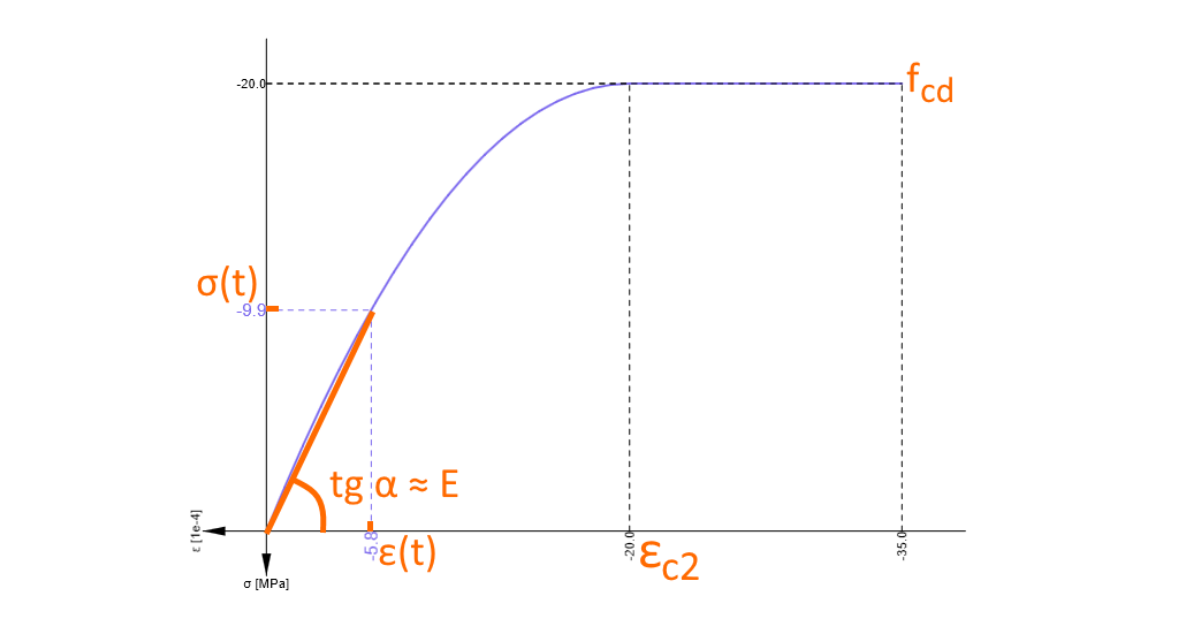# Calculation of stresses with elastic modulus E in ULS and SLS

$$Ultimate and serviceability limit state checks are based on different values of elastic modulus E.ULSElastic modulus is calculated from concrete stress value in specific age (introduce concrete compressive strength growth with age) and strain value at maximal strength values.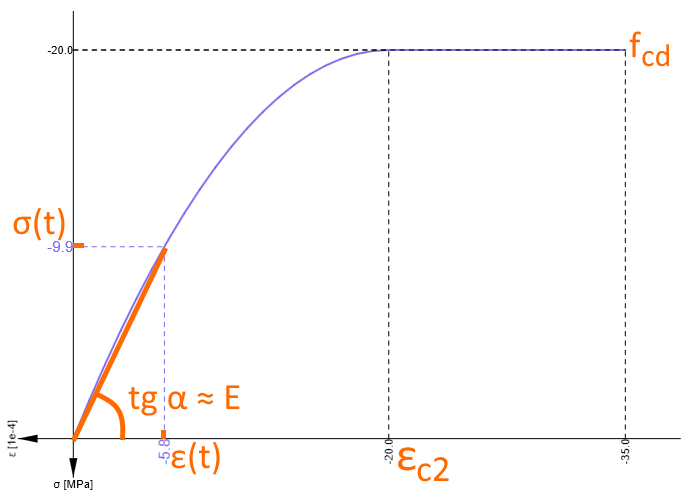The following table shows the growth of elastic modulus overtime in the last column, where after the age of 28 days the maximal value of 10GPa is reached.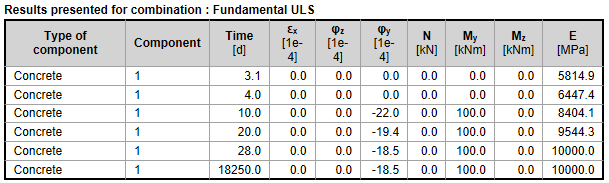SLSThe code considers Ecm value set for 0,4fcm as secant elastic modulus in chapter 3.1.3.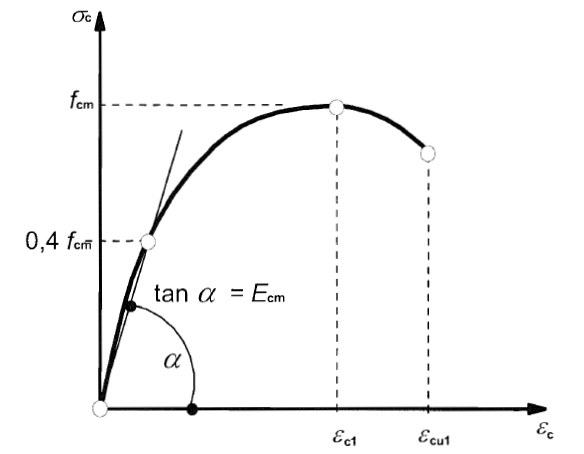In paragraph (3) it states: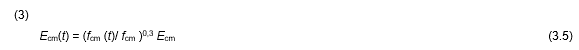Following table shows the growth of elastic modulus considered for characteristic combination (SLS):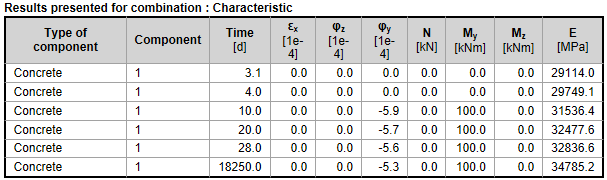The type of aggregate also changes the calculation of elastic modulus. The aggregate type used in concrete is set for Quarzite as a default value. The reduction of modulus is considered for limestone or sandstone aggregate and for basalt aggregate it applies the increase of elastic modulus.$$